## Introduction

Few challenges have drawn as much attention from a broad range of disciplines as the evolution of cooperative behaviour. From theoretical biology and behavioural ecology to economics, physics and mathematics, the study of the emergence of pro-social behaviour remains an open and demanding quest, both from experimental and theoretical points of view. In its most general flavour, cooperation dilemmas can be described as a conflict between individual and collective decisions. Take, for instance, the N-person Prisoner's dilemma (NPD) — the most common game metaphor for a Public Goods Game (PGG)1,2,3,4,5,6. Here, N players have the opportunity to contribute (or not) a certain amount c to a common pool (the “public good”). The sum of all contributions is invested (that is, it is multiplied by an enhancement factor F > 1) and the return is shared equally among all group members, irrespective of their contribution. From an individual (so-called rational) point of view, defecting or free-riding (not contributing) allows one to rip his/her share of the public good at no cost, to the extent that others help filling in the pot by contributing. Hence, defection emerges as the rational option, leading to the doomsday scenario of the tragedy of the commons2, reached when no one contributes and hence there are no resources to share.

Evolutionary game theory (EGT) posits this problem of cooperation by embedding it in a population and defining an evolutionary dynamics within that population of individuals. This formalism provides a natural and intuitive approach to many social and biological eco-systems. Here, the individual game payoff is typically associated with fitness or social success of an individual. The more successful (fitter) individuals will be imitated by others, so that the number of individuals adopting a given behaviour will evolve in time1,7. In a well-mixed scenario where each and every individual interacts equally likely with everyone else in the population and in the absence of additional mechanisms or stochastic effects, a population of Cooperators (Cs) and Defectors (Ds) will inevitably evolve towards the extinction of Cs. Hence, natural selection leads to the same outcome — the tragedy of the commons — as one expects from a group of rational players. This would happen despite the fact that all players would be better off with overall cooperation.

Several additional mechanisms have been proposed to resolve this paradoxical outcome, from decisions grounded in past encounters, to reputation-based strategies, risk aversion and punishment8,9,10,11,12,13,14,15,16,17,18. In particular, it is now well-known that, for both 2-person and N-person interactions, the structure of the population may play a determinant role in the evolution of a given behavior6,16,19,20,21,22,23,24,25,26,27,28,29. In a networked population, nodes typically represent individuals, whereas links represent shared goals, investments or exchanges. Irrespective of the way our social ties mold the overall population network, it is clear that the well-mixed assumption may only work in the limiting cases of small populations, as it is hardly conceivable that, in large populations, everyone can potentially interact with everyone else. Perhaps even more importantly, individuals may have a word on their interacting partners and with time decide who will be included in their list of peers. In other words, individuals are expected to be able to react against undesired partners, while striving to keep the desired ones, thereby modifying the social network in which they are embedded27,30,31,32,33,34,35,36,37,38,39. This problem can be addressed considering a dynamical social network. In the following, we study and build up the evolutionary dynamics of cooperation in a population of individuals playing PGGs on a network that may itself evolve as a result of the feedback received from the game interactions.

In a networked context, social neighbourhoods may represent individuals' local universes of partners with whom they can participate in social dilemmas of cooperation. Hence, whenever interacting in an N-person game, individuals will choose their partners from the pool of those they are connected with. In well-mixed populations, in turn, everyone shares the same set of potential partners, which corresponds to the entire population. In this context, well-mixed populations of players interacting in N-person dilemmas can be conveniently viewed as embedded in a fully connected (complete) interaction network (graph).

Let us add to such a setting the possibility that individuals reshape their set of acquaintances. Links may be broken at different rates, likely based on the type of link and its satisfiability6,31,32,40,41,42. Thus, in a world of Cs and Ds, we have three types of links — CC, CD and DD links — which we may characterize and differentiate, e.g., by attributing different break-up (γCC, γDC, γDD) and creation (αCC, αDC, αDD) rates, respectively31,32. Depending on the relative values of these rates and on the composition of the population, different network structures will emerge, with direct impact on each individual's neighbourhoods and ensuing returns from the games they play. These returns will dictate the evolution of strategies in a population which will change its composition and consequently will influence the network dynamics. In its full scope, this constitutes a tremendously hard problem. In the following section, we shall start by describing in detail a minimal model which, nonetheless, will allow us to gain and analyze key insights of such a co-evolutionary dynamics.

## Results

Let us consider a population of Z individuals, defined as nodes of a dynamical and bidirectional graph. Individuals interact through Public Goods Games (PGGs) played in groups of size N. Individuals setup groups by recruiting members of their neighbourhood (of size z > N), which thus constitutes the pool of potential co-group players.

The PGG described before has the structure of a NPD, such that the payoff of Cs and Ds in a group of size N in which k individuals play C and N-k individuals play D can be written as:where F < N ensures a NPD regime.

Let us now address network dynamics. We assume that individuals attempt to form new links at a constant rate α = αCC = αDC = αDD. However, the average lifetime of each link will depend on the strategy of the individuals it connects. In this way, we distinguish between CC, CD and DD links. Each of these will be broken at rates γCC, γDC, γDD, respectively. This “active linking” dynamics can be conveniently described by the following set of ordinary differential equations31,32:whereis the number of links between individuals of strategy l and m () andis the maximum possible number of these links. This maximum is frequency dependent, in the sense that it will depend on the number of individuals NI(t) and Nm(t) playing strategies l and m, respectively: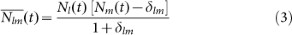If the characteristic timescale of the linking dynamics is much shorter than the time scale associated with strategy update (that is, the time that an individual takes to revise his/her strategy), the network will reach a steady state before the next strategy update takes place. That state is characterized by a stationary number of links given by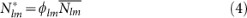whererepresents the fraction of active l-m links.

Assuming that NC(t) = k and ND(t) = Zk at a given steady state, we can compute the average number of links that any cooperator and defector will have:Substituting (4) in (5) we can express <zC> and <zD> as a function of the fraction of active links: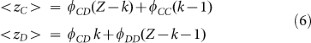The model description and calculations performed so far have been carried out in the mean-field approach. In keeping with this spirit, we now calculate the probability of having j cooperators in a group of size N, centred on a cooperator (HC(k,j)) or defector (HD(k,j)), respectively, whenever the composition of the population is characterized by k Cs and Z − k Ds. To this end, we employ a hypergeometric sampling10,12,43,44 and write:From these results we then determine the average fitness of strategies C and D by averaging the payoffs over all possible group compositions:Let us now describe how strategies evolve in time. We adopt a stochastic birth-death process combined with the pairwise comparison rule7, which accounts for both biological or cultural evolution. In a nutshell, in a biological setting, individuals with higher fitness will reproduce more often; in a cultural evolution setting, individuals with higher returns will tend to be imitated more often. To this end, in each time-step, a randomly chosen individual i1 will choose randomly another individual from the population i2. The former will imitate the latter with a probability p, which increases with the fitness difference. Here we adopt the Fermi distribution,, where β conveniently specifies the intensity of selection (β → 0 represents neutral drift andrepresents pure imitation dynamics)7,45.

In summary, for a finite population of size Z, at each birth-death event, there is a certain probability that a cooperator will change into a defector, T, or vice-versa, T+; they are given bywhereas the most probable direction of evolution, is given by the so-called gradient of selection, G(k) = T+(k) − T(k), a finite population analogue of the rate of change of Cs stemming from the replicator-like equation46 one obtains as Z → ∞. For finite Z, G(k) reads7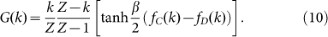Whenever G(k) > 0 (G(k) < 0), selection will act to increase (decrease) the number of Cs.

As discussed above, when individuals have enough time to adapt their social ties before deciding whether or not to change their behavioural strategy, then the system of ordinary differential equations which characterizes the linking dynamics may relax towards a steady state. Under such conditions we obtain a closed expression for the average fitness of Cs (fC) and Ds (fD). Clearly, this mean-field approach does not account for the fitness of each particular individual – it confers an average, population-wide, account of the evolution of each behaviour in the presence of a dynamical population structure.

In the absence of any network adaptation mechanism, Ds will benefit from higher returns when compared with Cs, making the gradient of selection (see eq. (10)) G(k) < 0, irrespectively of the fraction of Cs (k/Z). The same happens whenever links are randomly created and destroyed irrespectively of the strategies of the individuals located at the edges of those links. This can be realized with an adaptive network where γCC = γCD = γDD, shown in red in Fig. 1a. Alternatively, we may also consider a more realistic scenario in which the lifetime of a social link depends on the strategy of the individuals it connects. For instance, an individual may be dissatisfied with the return obtained from of her/his partners. This will typically happen whenever an individual is linked to a D, in the sense that it is counter-productive to anyone (being him a C or a D) to be connected (and therefore have him as a potential group member) with a free-rider.

Let us define a scenario, which we shall refer to in the following as Free Choice World (FCW), in which the linking dynamics is characterized by unilateral decisions regarding the break-up of links. In keeping with the previous discussion, this translates into γDD = γCD > γCC or, more conveniently, into γDD = γCD = εγCC, where ε ≥ 1 indicates how fast individuals react when they are linked to a defector. This leads to a different reshaping of the neighbourhoods, compared to the random situation described above, which, in turn, will act to change individuals' fitness. As a result, the impact of strategies on network dynamics leads now to a feedback onto the strategies, by changing their average fitness.

As shown in Fig. 1a, such network adaptation in group interactions creates an evolutionary dynamics scenario corresponding, at a population-wide level, to a new dilemma, characterized by two mixed internal equilibria, which were absent both in well-mixed populations and in the random case (see red line). Furthermore, this modification of the shape of the gradient of selection G transforms cooperation into a viable trait. While both Cs (below kL) and Ds (above kR) become disadvantageous when rare, the co-existence between Cs and Ds may become a finite population analogue (a probability attractor) of a stable fixed point in infinite populations at a frequency kR/Z. Hence, as long as cooperation initially prevails above a certain level kL, network adaptation in the framework of the FCW naturally leads to long periods of co-existence between Cs and Ds, rendering cooperation viable.

Let us investigate the roots of G(k). This is equivalent to determining the roots of the fitness difference,, which, after some algebra becomes: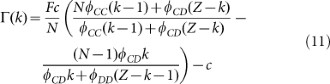Eq. (11) is a second order polynomial in k, which means thathas between zero and two (real) internal roots (in the interval 0 < k < Z). Their associated stability will be determined by the signs ofand: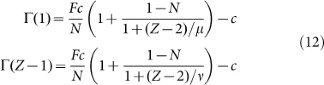Whereand.

Given that Z > N > 1 and whenever F < N – such that individuals play a NPD, it is easy to verify thatand. Thus, we will either have zero or two internal roots, given the order of the polynomial in Eq. (11). In other words we can either have an effective defection dominance game — negative gradient for all values of k — or an N-person coordination dilemma43, where the gradient of selection shows both coordination (kL) and coexistence (kR) equilibria, with. Differently, for F > N — where cooperation always prevails in well-mixed populations — the situation will be reversed, that is,and, in certain cases. For a given μ and, there is a critical multiplication factor,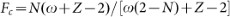, with, above which the gradient will be reversed, i.e., we either have a harmony game or a reversed N-person coordination game with.

The results shown in Fig. 1b confirm these features and concern the most intereresting case of F > N. For each value of F, one can draw a horizontal line that defines the location of the internal roots at the intersection with the curves drawn: one unstable root (kL) and another stable root (kL < kR). Interestingly, with the increase of the break-up rate (ε), not only we observe an increase in the critical number of Cs (kL) necessary to reach a stable cooperative basin of attraction, but also we obtain an increase of the stable fraction of Cs where the population will spend most of its time, once this basin is reached. Fig. 1b also shows that only 2 types of dynamical scenarios can appear: For large multiplication factors F (F →N), the above combination involving 2 internal fixed points emerges, whereas the distinctive defection dominance dilemma usually associated with the NPD is recovered for low values of F (as F →1). It is noteworthy that these results remain valid under a more conventional description of evolutionary dynamics associated with the replicator-like dynamics equation and infinite populations (by taking the limit, see Eq. (10)) – hence our application of nomenclature (derived from deterministic dynamical systems) to stochastic evolutionary dynamics.

Up to now, link break-up was taken to constitute a unilateral decision. In reality, one can imagine situations in which a connection is broken by mutual consent or as a result of some sort of negotiation towards conflict resolution, which will naturally create an effective break-up rate of links. Specific values will depend on the situation, but in general one may safely assume that. Hence, in such a Bounded Choice World (BCW), the lifetime of links connecting two Ds will most likely be shorter than the lifetime of links connecting a C and a D (in the sense that it is crucial for a free-rider to have a C in the group, but not additional free-riders). A simple realization of this scenario is obtained assuming, as before γDD = εγCC and taking γCD = (γCC + γDD)/2. As shown in Fig. 2, this variation with respect to the simpler FCW shown before, does not change the nature of the effective dilemma one obtains at a population level.

### Tunneling towards a cooperative basin

Analysis of the gradient of selection provides an overview of the population-wide dynamics. This is particularly relevant, given the coordination barrier, which divides the system in two basins of attraction. Classically one uses the fixation probability to calculate the chance of a single C or D to spread and completely invade a population of players using the opposite strategy47. However, the existence of stable equilibria may render such an analysis of the fixation probability misleading, as the time required for the population to reach any monomorphic state may become arbitrarily long48, in particular whenever G(k) exhibits basins of attraction to stable polymorphic configurations. Furthermore, if one considers other forms of stochastic effects, such as random exploration of strategies49 the system will ne3)ver actually fixate.

A convenient alternative which overcomes the drawbacks of the fixation probability, consists in the analysis of the stationary distributions P(k/Z)50 of the complete Markov chain (of size Z + 1), defined by the transition probabilities to increase and decrease the number of cooperators. To this end, it is convenient to assume that, besides imitating the actions of the most successful individuals, individuals may explore the available spectrum of strategies with a given (mutation) probability μ49.

The probabilities entering the tridiagonal transition matrixof the Markov chain are defined asand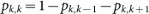, wherestand for the transition probabilities computed for an arbitrary mutation probability μ, which are given byfor the probability to increase from k to k + 1 Cs andfor the probability to decrease from k to k − 1 Cs. The stationary distribution is then obtained, as usual, from the eigenvector corresponding to the eigenvalue 1 of S51,52.

Fig. 3a shows the stationary distribution — i.e., the prevalence in time of a given configuration of the population specified by the fraction of cooperators — for the FCW, adopting the same parameters of Fig. 1, with μ = 1/Z. As expected, for ε = 1, the population spends most of the time in the vicinity of the k = 0 configuration, as we have a traditional defection dominant dilemma. Yet, as we increase the rate at which individuals react to adverse ties (ε), we observe the emergence of a single peak near the coexistence equilibrium. Hence, regardless of the initial condition, the population is able to profit from the stochastic effects — resulting both from imitation errors and exploration dynamics — to tunnel through the coordination barrier and spend most of its time at a co-existence configuration in which cooperators prevail.